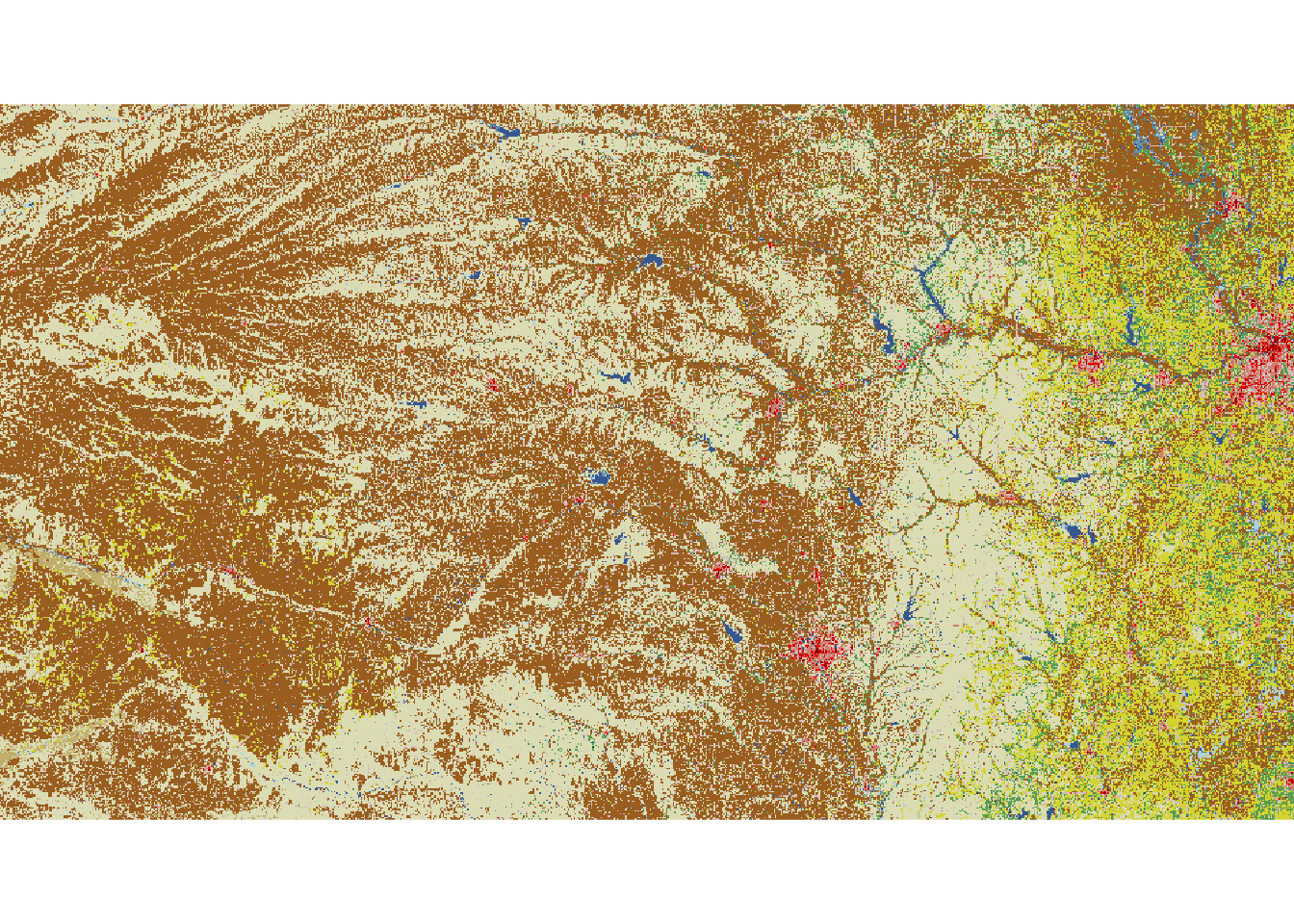# 8 September 10

## 8.1 Announcements

• No new announcements today

## 8.2 Extreme precipitation in Kansas

• During the next few lectures, I will demonstrate multiple ways that I would go about meeting the goals in assignment #2
• My process
• Determine the goals of the study
• Exploratory data analysis
• Live demonstration
• The model building process
• 1). Choose appropriate PDFs or PMFs for the data, process, and parameter models
• 2). Choose appropriate mathematical models for the “parameters” or moments of the PDFs/PMFs from step 1.
• 3). Choose an algorithm fit the statistical model to the data
• 4). Make statistical inference (e.g., calculate derived quantities and summarize the posterior distribution)
• Model checking, improvements, validation, and selection (Ch. 6)
• What we will need to learn
• How to use R as a geographic information system
• How to use the hierarchical modeling framework to describe Kriging
• Hierarchical Bayesian model vs. “empirical” hierarchical model
• Specialized language used in spatial statistics (e.g., range, nugget, variogram)
• New general tools from statistics
• Gaussian process
• Metropolis and Metropolis–Hastings algorithms
• Gibbs sampler

## 8.3 Intro to GIS

• Spatio-temporal data from a statistical and GIS perspective are quite different
• Both disciplines, however, usually classify data based on the spatial support of the “data”
• Data from the GIS perspective
• Using R as a GIS, there are four main types of “data” that we will use.
• Shapefiles
• Raster
• Points

### 8.3.1 Shapefiles

• Shapefiles are generally used to represent continuous spatial objects and boundaries
• Examples
• Example: Shapefiles of each state from the census website

library(rgdal)

url <- "http://www2.census.gov/geo/tiger/GENZ2015/shp/cb_2015_us_state_5m.zip"

tmpdir <- tempdir()
file <- basename(url)
unzip(file, exdir = tmpdir)
shapefile <- paste(tmpdir, "/cb_2015_us_state_5m.shp", sep = "")
ogrListLayers(shapefile)
sf.usa <- readOGR(shapefile, layer = "cb_2015_us_state_5m")

sf.kansas <- sf.usa[which(sf.usa@data\$NAME == "Kansas"), ]
plot(sf.kansas)

### 8.3.2 Raster

• Rasters are geographically referenced images (i.e., discrete spatial data)
• Examples
• Raster files can be very large (e.g., NLCD is 1.1 Gb compressed and 18 Gb uncompressed)
• Raster “data” are usually model based predictions (e.g., PRISM) -Example: 2011 NLCD
library(raster)

# Large file that you may want to save on your computer
url.nlcd <- "https://www.dropbox.com/s/rc51iepbo8d3auk/KS_2011_NLCD.img?dl=1"
setwd("~/Google Drive/Teaching/Fall 2020/STAT 764 (F2020)/STAT 764 Lecture Notes/Large data sets")
url.nlcd <- "KS_2011_NLCD.img"
rl.nlcd2011 <- raster(url.nlcd)

plot(rl.nlcd2011)
library(raster)
## Loading required package: sp
# Large file that you may want to save on your computer
url.nlcd <- "https://www.dropbox.com/s/rc51iepbo8d3auk/KS_2011_NLCD.img?dl=1"
setwd("~/Google Drive/Teaching/Fall 2020/STAT 764 (F2020)/STAT 764 Lecture Notes/Large data sets")
url.nlcd <- "KS_2011_NLCD.img"
rl.nlcd2011 <- raster(url.nlcd)

plot(rl.nlcd2011)• Transformations and coordinate reference systems
• Not all spatial files have the same coordinate reference systems.
sf.kansas <- spTransform(sf.kansas, crs(rl.nlcd2011))

plot(rl.nlcd2011)
plot(sf.kansas, add = TRUE, lwd = 4)

### 8.3.3 Points

• Point files are created from coordinates (and a corresponding coordinate system)
• Example: Plot location of Dickens Hall
pt.dickens <- data.frame(long = -96.579382, lat = 39.190433)  #Location of Dickens Hall
coordinates(pt.dickens) = ~long + lat
proj4string(pt.dickens) <- CRS("+proj=longlat +datum=WGS84 +no_defs +ellps=WGS84 +towgs84=0,0,0")
pt.dickens <- spTransform(pt.dickens, crs(rl.nlcd2011))

plot(rl.nlcd2011)
plot(sf.kansas, add = TRUE, lwd = 4)
plot(pt.dickens, add = TRUE, col = "green", pch = 20, cex = 3)
• Get the landcover type at this location

extract(rl.nlcd2011, pt.dickens)  # See legend at https://www.mrlc.gov/data/legends/national-land-cover-database-2011-nlcd2011-legend

## 8.4 Summary

• There are entire courses on what we covered today
• This is an area that is rapidly developing